# Physics investigatory board project ON TRANSFORMER

21 de Apr de 2020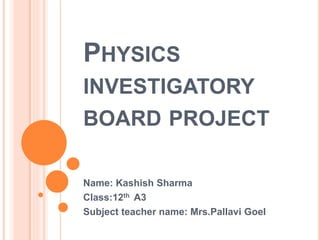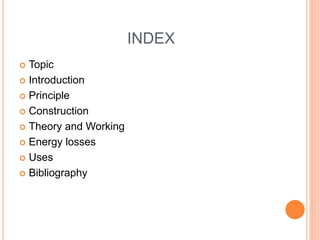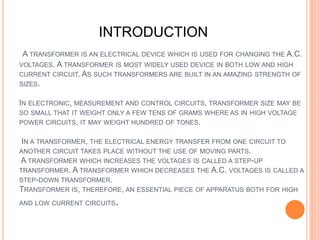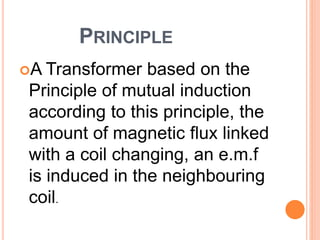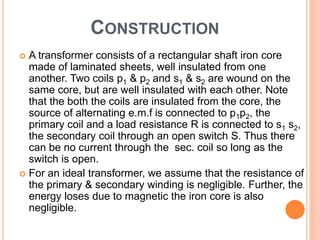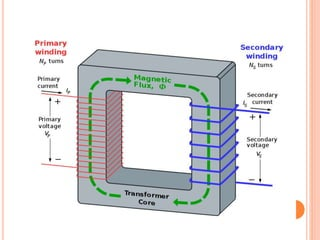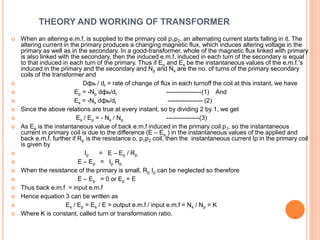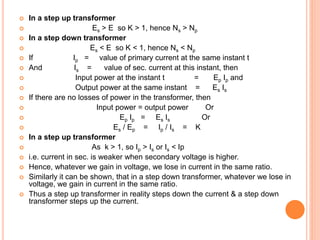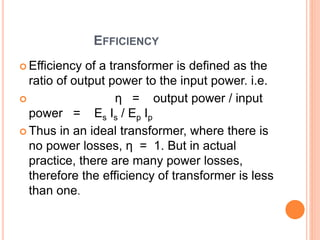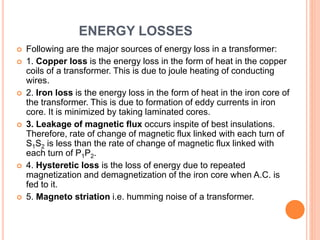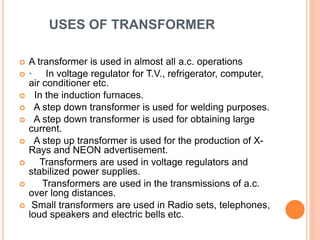1 de 14

### Physics investigatory board project ON TRANSFORMER

• 1. PHYSICS INVESTIGATORY BOARD PROJECT Name: Kashish Sharma Class:12th A3 Subject teacher name: Mrs.Pallavi Goel
• 2.  I Kashish Sharma of class XII- A 3,Of DPSG Meerut Road, would like to express my special thanks of gratitude to my teacher Mrs. Pallavi Goel as well as our principal Mrs. Jyoti Gupta who gave me the golden opportunity to do this wonderful project on the topic :Transformer and its principle, which also helped me in doing a lot of Research and I came to know about so many new things I am really thankful to them .  Secondly, I would also like to thank my parents and friends who helped me a lot in finalizing this project within the limited frame of time . 
• 3. INDEX  Topic  Introduction  Principle  Construction  Theory and Working  Energy losses  Uses  Bibliography
• 5. A TRANSFORMER IS AN ELECTRICAL DEVICE WHICH IS USED FOR CHANGING THE A.C. VOLTAGES. A TRANSFORMER IS MOST WIDELY USED DEVICE IN BOTH LOW AND HIGH CURRENT CIRCUIT. AS SUCH TRANSFORMERS ARE BUILT IN AN AMAZING STRENGTH OF SIZES. IN ELECTRONIC, MEASUREMENT AND CONTROL CIRCUITS, TRANSFORMER SIZE MAY BE SO SMALL THAT IT WEIGHT ONLY A FEW TENS OF GRAMS WHERE AS IN HIGH VOLTAGE POWER CIRCUITS, IT MAY WEIGHT HUNDRED OF TONES. IN A TRANSFORMER, THE ELECTRICAL ENERGY TRANSFER FROM ONE CIRCUIT TO ANOTHER CIRCUIT TAKES PLACE WITHOUT THE USE OF MOVING PARTS. A TRANSFORMER WHICH INCREASES THE VOLTAGES IS CALLED A STEP-UP TRANSFORMER. A TRANSFORMER WHICH DECREASES THE A.C. VOLTAGES IS CALLED A STEP-DOWN TRANSFORMER. TRANSFORMER IS, THEREFORE, AN ESSENTIAL PIECE OF APPARATUS BOTH FOR HIGH AND LOW CURRENT CIRCUITS. INTRODUCTION
• 6. PRINCIPLE A Transformer based on the Principle of mutual induction according to this principle, the amount of magnetic flux linked with a coil changing, an e.m.f is induced in the neighbouring coil.
• 7. CONSTRUCTION  A transformer consists of a rectangular shaft iron core made of laminated sheets, well insulated from one another. Two coils p1 & p2 and s1 & s2 are wound on the same core, but are well insulated with each other. Note that the both the coils are insulated from the core, the source of alternating e.m.f is connected to p1p2, the primary coil and a load resistance R is connected to s1 s2, the secondary coil through an open switch S. Thus there can be no current through the sec. coil so long as the switch is open.  For an ideal transformer, we assume that the resistance of the primary & secondary winding is negligible. Further, the energy loses due to magnetic the iron core is also negligible.
• 9. THEORY AND WORKING OF TRANSFORMER  When an altering e.m.f. is supplied to the primary coil p1p2, an alternating current starts falling in it. The altering current in the primary produces a changing magnetic flux, which induces altering voltage in the primary as well as in the secondary. In a good-transformer, whole of the magnetic flux linked with primary is also linked with the secondary, then the induced e.m.f. induced in each turn of the secondary is equal to that induced in each turn of the primary. Thus if Ep and Es be the instantaneous values of the e.m.f.’s induced in the primary and the secondary and Np and Ns are the no. of turns of the primary secondary coils of the transformer and  Dфь / dt = rate of change of flux in each turnoff the coil at this instant, we have  Ep = -Np dфь/dt -----------------(1) And  Es = -Ns dфь/dt ----------------- (2)  Since the above relations are true at every instant, so by dividing 2 by 1, we get  Es / Ep = - Ns / Np ----------------(3)  As Ep is the instantaneous value of back e.m.f induced in the primary coil p1, so the instantaneous current in primary coil is due to the difference (E – Ep ) in the instantaneous values of the applied and back e.m.f. further if Rp is the resistance o, p1p2 coil, then the instantaneous current Ip in the primary coil is given by  Ip = E – Ep / Rp  E – Ep = Ip Rp  When the resistance of the primary is small, Rp Ip can be neglected so therefore  E – Ep = 0 or Ep = E  Thus back e.m.f = input e.m.f  Hence equation 3 can be written as  Es / Ep = Es / E = output e.m.f / input e.m.f = Ns / Np = K  Where K is constant, called turn or transformation ratio.
• 10.  In a step up transformer  Es > E so K > 1, hence Ns > Np  In a step down transformer  Es < E so K < 1, hence Ns < Np  If Ip = value of primary current at the same instant t  And Is = value of sec. current at this instant, then  Input power at the instant t = Ep Ip and  Output power at the same instant = Es Is  If there are no losses of power in the transformer, then  Input power = output power Or  Ep Ip = Es Is Or  Es / Ep = Ip / Is = K  In a step up transformer  As k > 1, so Ip > Is or Is < Ip  i.e. current in sec. is weaker when secondary voltage is higher.  Hence, whatever we gain in voltage, we lose in current in the same ratio.  Similarly it can be shown, that in a step down transformer, whatever we lose in voltage, we gain in current in the same ratio.  Thus a step up transformer in reality steps down the current & a step down transformer steps up the current.
• 11. EFFICIENCY  Efficiency of a transformer is defined as the ratio of output power to the input power. i.e.  η = output power / input power = Es Is / Ep Ip  Thus in an ideal transformer, where there is no power losses, η = 1. But in actual practice, there are many power losses, therefore the efficiency of transformer is less than one.
• 12. ENERGY LOSSES  Following are the major sources of energy loss in a transformer:  1. Copper loss is the energy loss in the form of heat in the copper coils of a transformer. This is due to joule heating of conducting wires.  2. Iron loss is the energy loss in the form of heat in the iron core of the transformer. This is due to formation of eddy currents in iron core. It is minimized by taking laminated cores.  3. Leakage of magnetic flux occurs inspite of best insulations. Therefore, rate of change of magnetic flux linked with each turn of S1S2 is less than the rate of change of magnetic flux linked with each turn of P1P2.  4. Hysteretic loss is the loss of energy due to repeated magnetization and demagnetization of the iron core when A.C. is fed to it.  5. Magneto striation i.e. humming noise of a transformer.
• 13. USES OF TRANSFORMER  A transformer is used in almost all a.c. operations  · In voltage regulator for T.V., refrigerator, computer, air conditioner etc.  In the induction furnaces.  A step down transformer is used for welding purposes.  A step down transformer is used for obtaining large current.  A step up transformer is used for the production of X- Rays and NEON advertisement.  Transformers are used in voltage regulators and stabilized power supplies.  Transformers are used in the transmissions of a.c. over long distances.  Small transformers are used in Radio sets, telephones, loud speakers and electric bells etc.
• 14. BIBLIOGRAPHY  PHYSICS CLASS 12TH NCERT TEXTBOOK  www.yahoo.com  www.google.com  www.live.com  www.rediffmail.com